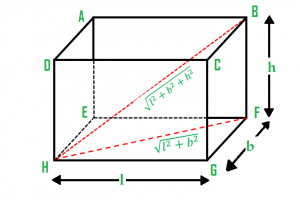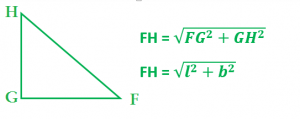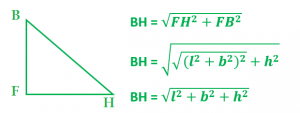# Length of longest rod that can fit into a cuboid

• Last Updated : 09 Nov, 2021

Given the length, breadth, and height of a cuboid, the task is to find the length of the longest rod that can fit in a cuboid.
Examples:

```Input: length = 12, breadth = 9, height = 8
Output: 17

Input: length = 22, breadth = 19, height = 8
Output: 30.1496```Explanation: In the figure

`GH = length, GF = breadth, FB= height`

The length of the longest rod is BH. Therefore to compute BH we can apply Pythagoras Theorem in Triangle BHF. From triangle HGF we can compute the length of FH.After getting the length of FH we can find the length of BH using Pythagoras theorem in triangle BHF.Below is the implementation of the above approach:

## C++

 `// C++ program to find the longest rod``// that can fit in a cuboid``#include ``using` `namespace` `std;` `// Function to find the length``double` `longestRodInCuboid(``int` `length,``                        ``int` `breadth, ``int` `height)``{``    ``double` `result;``    ``int` `temp;` `    ``// temporary variable to hold``    ``// the intermediate result``    ``temp = length * length + breadth * breadth``           ``+ height * height;` `    ``// length of longest rod is calculated``    ``// using square root function``    ``result = ``sqrt``(temp);` `    ``return` `result;``}` `// Driver code``int` `main()``{``    ``int` `length = 12, breadth = 9, height = 8;` `    ``// calling longestRodInCuboid() function to``    ``// get the length of longest rod``    ``cout << longestRodInCuboid(length, breadth, height);` `    ``return` `0;``}`

## Java

 `// Java program to find the longest``// rod that can fit in a cuboid``class` `GFG``{` `// Function to find the length``static` `double` `longestRodInCuboid(``int` `length,``                                 ``int` `breadth,``                                 ``int` `height)``{``    ``double` `result;``    ``int` `temp;``    ` `    ``// temporary variable to hold``    ``// the intermediate result``    ``temp = length * length + breadth *``           ``breadth + height * height;``    ` `    ``// length of longest rod is calculated``    ``// using square root function``    ``result = Math.sqrt(temp);``    ` `    ``return` `result;``}` `// Driver Code``public` `static` `void` `main(String[] args)``{``    ``int` `length = ``12``,``        ``breadth = ``9``,``        ``height = ``8``;` `    ``// calling longestRodInCuboid()``    ``// function to get the length``    ``// of longest rod``    ``System.out.println((``int``)longestRodInCuboid(length,``                                    ``breadth, height));``}``}` `// This code is contributed by ChitraNayal`

## Python 3

 `# Python 3 program to find the longest rod``# that can fit in a cuboid` `# from math lib. import everything``from` `math ``import` `*` `# Function to find the length``def` `longestRodInCuboid(length, breadth, height) :` `    ``# temporary variable to hold``    ``# the intermediate result``    ``temp ``=` `length ``*` `length ``+` `breadth  ``*` `breadth ``+` `height ``*` `height` `    ``#  length of longest rod is calculated``    ``# using square root function``    ``result ``=` `sqrt(temp)` `    ``return` `result`  `# Driver Code``if` `__name__ ``=``=` `"__main__"` `:` `    ``length, breadth, height ``=` `12``, ``9``, ``8` `    ``# calling longestRodInCuboid() function to``    ``# get the length of longest rod``    ``print``(longestRodInCuboid(length, breadth, height))`  `# This code is contributed by ANKITRAI1`

## C#

 `// C# program to find the longest``// rod that can fit in a cuboid``using` `System;``class` `GFG``{` `// Function to find the length``static` `double` `longestRodInCuboid(``int` `length,``                                ``int` `breadth,``                                ``int` `height)``{``    ``double` `result;``    ``int` `temp;``    ` `    ``// temporary variable to hold``    ``// the intermediate result``    ``temp = length * length + breadth *``        ``breadth + height * height;``    ` `    ``// length of longest rod is calculated``    ``// using square root function``    ``result = Math.Sqrt(temp);``    ` `    ``return` `result;``}` `// Driver Code``public` `static` `void` `Main()``{``    ``int` `length = 12,``        ``breadth = 9,``        ``height = 8;` `    ``// calling longestRodInCuboid()``    ``// function to get the length``    ``// of longest rod``    ``Console.WriteLine((``int``)longestRodInCuboid(length,``                                    ``breadth, height));``}``}` `// This code is contributed by inder_verma..`

## PHP

 ``

## Javascript

 ``

Output:

`17`

Time Complexity: O(1)

Auxiliary Space: O(1)

My Personal Notes arrow_drop_up